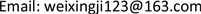1. 引言

2. 研究概述

2.1. 收集数据

2.2. 分析呈现数据

1、[18,20]1，[21,23]=2，[24,26]=3

2、[0,33]=1，[34,66]=2，[67,100]=3

3、[0,3000]=1，[3000,4500]=2，[4500,6000]=3

4、=1，=2，=3

5、[0,8]=1，[8,10]=2，[10,12]=3

2.3. 可视化分析预测

Apriori算法  是常用的用于挖掘出数据关联规则的算法，它用来找出数据值中频繁出现的数据集合，找出这些集合的模式有助于我们做一些决策。常用的频繁项集的评估标准有支持度，置信度和提升度三个。

Support ( X , Y ) = P ( X , Y ) = number ( XY ) / num ( AllSamples ) (1)

Support ( X , Y , Z ) = P ( X , Y , Z ) = number ( XYZ ) / num ( AllSamples ) (2)

Confidence ( X ⇐ Y ) = P ( Z | Y ) = P ( XY ) / P ( Y ) (3)

Confidence ( X ⇐ YZ ) = P ( X | YZ ) = P ( XYZ ) / P ( YZ ) (4)

Lift ( X ⇐ Y ) = P ( X | Y ) / P ( X ) = Confidence ( X ⇐ Y ) / P ( X ) (5)

3. 结束语

985高校，六级，农村 7~10 k，一线城市

985高校，六级，城市 5~7 k，二线城市

1) 985高校毕业生对薪资要求较高，更倾向于一线城市

2) 专业不是影响就业的主要因素

3) 城市生源的毕业生倾向于二线城市，对薪资最低接受限度较低

1) 五百强企业主要分布在一线及海外城市，且工资普遍较高。最看重毕业生的条件是毕业院校和外语水平，要求偏高。

2) 国企和私企大多分布在二线和一线城市，对毕业生的要求院校依旧偏高，但不高于五百强企业，对外语水平的要求比较宽松。

3) 家庭条件不是影响就业的主要因素。

1) 毕业生和企业在高校、外语等方面的供需关系是比较平衡的。

2) 毕业生和企业在薪资方面有一定矛盾。毕业生需求较高，企业提供的较低。

2018年度河南省大中专院校就业创业研究立项课题JYB2018074。